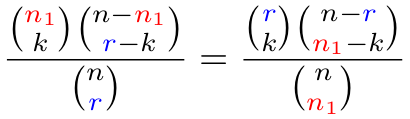# Hypergeometric distribution symmetry

One of these days I’d like to read Feller’s probability book slowly. He often says clever things in passing that are easy to miss.

Here’s an example from Feller  that I overlooked until I saw it cited elsewhere.

Suppose an urn contains n marbles, n1 red and n2 black. When r marbles are drawn from the urn, the probability that precisely k of the marbles are red follows a hypergeometric distribution.Feller mentions that this expression can be rewritten asWhat just happened? The roles of the total number of red marbles n1 and the sample size r, have been swapped.

The proof is trivial—expand both expressions using factorials and observe that they’re equal—but that alone doesn’t give much insight. It just shows that two jumbles of symbols represent the same quantity.

The combinatorial interpretation is more interesting and more useful. It says that you have two ways to evaluate the probability: focusing on the sample or focusing on the population of red marbles.

The former perspective says we could multiply the number of ways to choose k marbles from the supply of n1 red marbles and rk black marbles from the supply of nn1 black marbles, then divide by the number of ways to choose a sample of r marbles from an urn of n marbles.

The latter perspective says that rather than partitioning our sample of r marbles, we could think of partitioning the population of red marbles into two groups: those included in our sample and those not in our sample.

I played around with adding color to make it easier to see how the terms move between the two expressions. I colored the number of red marbles red and the sample size blue.Moving from left to right, the red variable goes down and the blue variable goes up.

## Related posts

 William Feller. An Introduction to Probability Theory and Its Applications. Volume 1. Third edition. Page 44.